Publicité

# CS767_Lecture_05.pptx

5 Sep 2022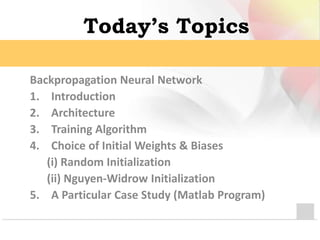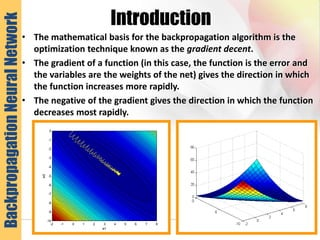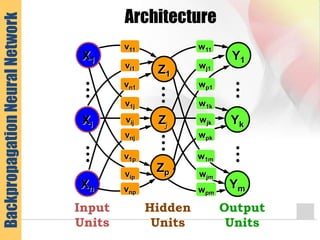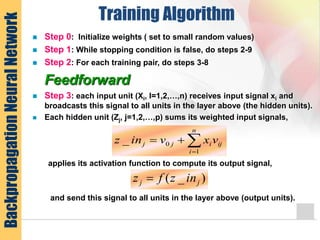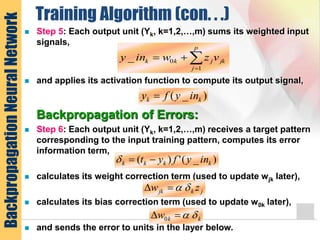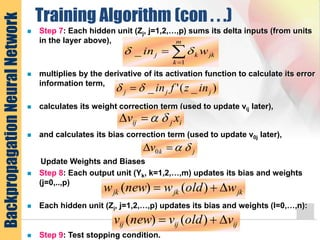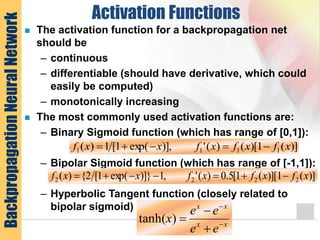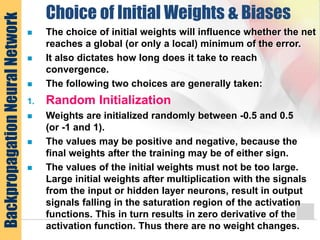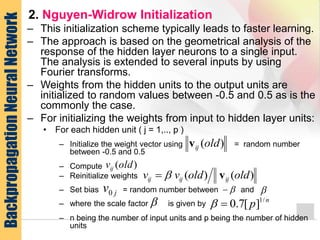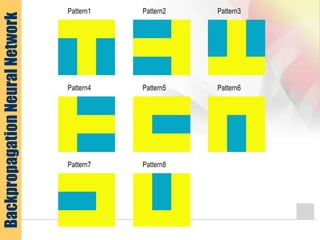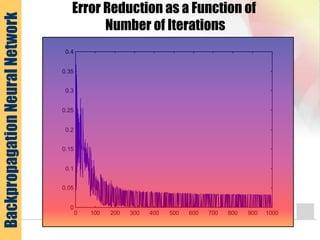1 sur 14
Publicité

### CS767_Lecture_05.pptx

1. Advance Topics in Artificial Intelligence CSC 767 Learning Algorithm - Artificial Neural Networks (ANN) Courtesy Shahid Abid
2. Today’s Topics Backpropagation Neural Network 1. Introduction 2. Architecture 3. Training Algorithm 4. Choice of Initial Weights & Biases (i) Random Initialization (ii) Nguyen-Widrow Initialization 5. A Particular Case Study (Matlab Program)
3. Introduction • The mathematical basis for the backpropagation algorithm is the optimization technique known as the gradient decent. • The gradient of a function (in this case, the function is the error and the variables are the weights of the net) gives the direction in which the function increases more rapidly. • The negative of the gradient gives the direction in which the function decreases most rapidly. -2 -1 0 1 2 3 4 5 6 7 8 -10 -9 -8 -7 -6 -5 -4 -3 -2 -1 0 w1 w2 Backpropagation Neural Network
4. Architecture l l l Hidden Units l l l Z1 Zj Zp Output Units Y1 l l l Yk l l l Ym Input Units l l l l l l Xi X1 Xn v11 vi1 vn1 v1j vij vnj v1p vip vnp w11 wj1 wp1 w1k wjk wpk w1m wjm wpm Backpropagation Neural Network
5. Training Algorithm  Step 0: Initialize weights ( set to small random values)  Step 1: While stopping condition is false, do steps 2-9  Step 2: For each training pair, do steps 3-8 Feedforward  Step 3: each input unit (Xi, I=1,2,…,n) receives input signal xi and broadcasts this signal to all units in the layer above (the hidden units).  Each hidden unit (Zj, j=1,2,…,p) sums its weighted input signals, applies its activation function to compute its output signal, and send this signal to all units in the layer above (output units).     n i ij i j j v x v in z 1 0 _ ) _ ( j j in z f z  Backpropagation Neural Network
6. Training Algorithm (con. . .)  Step 5: Each output unit (Yk, k=1,2,…,m) sums its weighted input signals,  and applies its activation function to compute its output signal, Backpropagation of Errors:  Step 6: Each output unit (Yk, k=1,2,…,m) receives a target pattern corresponding to the input training pattern, computes its error information term,  calculates its weight correction term (used to update wjk later),  calculates its bias correction term (used to update w0k later),  and sends the error to units in the layer below.     p j jk j k k v z w in y 1 0 _ ) _ ( k k in y f y  ) _ ( ' ) ( k k k k in y f y t    j k jk z w     k k w     0 Backpropagation Neural Network
7. Training Algorithm (con . . .)  Step 7: Each hidden unit (Zj, j=1,2,…,p) sums its delta inputs (from units in the layer above),  multiplies by the derivative of its activation function to calculate its error information term,  calculates its weight correction term (used to update vij later),  and calculates its bias correction term (used to update v0j later), Update Weights and Biases  Step 8: Each output unit (Yk, k=1,2,…,m) updates its bias and weights (j=0,..,p)  Each hidden unit (Zj, j=1,2,…,p) updates its bias and weights (I=0,…,n):  Step 9: Test stopping condition. i j ij x v     j k v     0 ) _ ( ' _ j j j in z f in       m k jk k j w in 1 _   jk jk jk w old w new w    ) ( ) ( ij ij ij v old v new v    ) ( ) ( Backpropagation Neural Network
8. Activation Functions  The activation function for a backpropagation net should be – continuous – differentiable (should have derivative, which could easily be computed) – monotonically increasing  The most commonly used activation functions are: – Binary Sigmoid function (which has range of [0,1]): – Bipolar Sigmoid function (which has range of [-1,1]): – Hyperbolic Tangent function (closely related to bipolar sigmoid) )] ( 1 )[ ( ) ( ' )], exp( 1 [ 1 ) ( 1 1 1 1 x f x f x f x x f      )] ( 1 )][ ( 1 [ 5 . 0 ) ( ' , 1 )]} exp( 1 [ 2 { ) ( 2 2 2 2 x f x f x f x x f        x x x x e e e e x      ) tanh( Backpropagation Neural Network
9. Choice of Initial Weights & Biases  The choice of initial weights will influence whether the net reaches a global (or only a local) minimum of the error.  It also dictates how long does it take to reach convergence.  The following two choices are generally taken: 1. Random Initialization  Weights are initialized randomly between -0.5 and 0.5 (or -1 and 1).  The values may be positive and negative, because the final weights after the training may be of either sign.  The values of the initial weights must not be too large. Large initial weights after multiplication with the signals from the input or hidden layer neurons, result in output signals falling in the saturation region of the activation functions. This in turn results in zero derivative of the activation function. Thus there are no weight changes. Backpropagation Neural Network
10. 2. Nguyen-Widrow Initialization – This initialization scheme typically leads to faster learning. – The approach is based on the geometrical analysis of the response of the hidden layer neurons to a single input. The analysis is extended to several inputs by using Fourier transforms. – Weights from the hidden units to the output units are initialized to random values between -0.5 and 0.5 as is the commonly the case. – For initializing the weights from input to hidden layer units: • For each hidden unit ( j = 1,.., p ) – Initialize the weight vector using = random number between -0.5 and 0.5 – Compute – Reinitialize weights – Set bias = random number between and – where the scale factor is given by – n being the number of input units and p being the number of hidden units ) (old vij ) (old ij v ) ( ) ( old old v v ij ij ij v   j v0     n p / 1 ] [ 7 . 0   Backpropagation Neural Network
11. Backpropagation Neural Network
12. Backpropagation Neural Network Error Reduction as a Function of Number of Iterations 0 100 200 300 400 500 600 700 800 900 1000 0 0.05 0.1 0.15 0.2 0.25 0.3 0.35 0.4
Publicité